# Bricks II

3/4 bricks weighs 6 kg and 2/3 bricks. How weighs one whole brick?

Result

x =  72 kg

#### Solution:

3/4x = 6+ 2/3 x

x = 72

x = 72

Calculated by our simple equation calculator.

Leave us a comment of example and its solution (i.e. if it is still somewhat unclear...):Be the first to comment!#### To solve this example are needed these knowledge from mathematics:

Do you have a linear equation or system of equations and looking for its solution? Or do you have quadratic equation?

## Next similar examples:

1. Bob and Bobek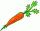Bobek has 2 cabbages and 5 carrots, Bob has 2 cabbages and 9 carrots. When Bobek stepped on the scale weight 5800 g and when Bob weight 8960 g. Actual Bob weight is 8 kg and Bobek 5 kg. How much weighs one carrot?
2. Bag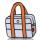Nelly found interesting point. An empty bag weighs 4 kg less than full. And empty bag is 5 times easier than full. How many kg weigh things in this bag?
3. Sumo competition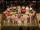In the competition for the heaviest man was 5 competitors. The first three together weighed 553 kg. What is the minimal weigt of winner, if a contestant weighed with an accuracy of one kilogram.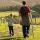Daddy is a 46 year old and son is at age of 16. When (which year) was/will daddy 5 times more years than his son?
5. Apples 3Julka has 5 apples more than Hugo and four apples less than Annie. Hugo has 17 apples. How many apples has Julka and how Annie?
6. Class alphabet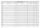All 29 pupils in the class are written in a class by alphabet. The number of pupils enrolled before Paul is three times higher than the number of pupils behind him. Calculate how many pupils are enrolled after Paul.
7. Chewing gums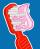For 3 chewing gums, you will pay 20 CZK less than 7 chewing gums. How much is 1 chewing gum and how much does a 5pcs package cost?
8. If-then equationIf 5x - 17 = -x + 7, then x =
9. Six te 2If 3t-7=5t, then 6t=
10. Eq1Solve equation: 4(a-3)=3(2a-5)
11. PopsiclesFrancis went to buy ice lollies. If he buy 8 popsicles he missed 4 USD. When he buy 7 popsicles, got back 1 USD. How many USD was a popsicle?
12. Forest nurseryIn the forest nursery after winter, they found that 1/10 stems died out of them. For them, they land 193 new spruces. How many spruces are in the forest nursery?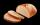Several pieces of bread were in the store. Half of them plus a half of bread bought a cookbook. Of the remaining bread, half of it plus half of the bread was bought by Mr. Novák. The last bread was bought by Ms. Small. How many breads were in the store at.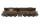Daily passes the same track section 8 pairs of trains with average 2400 passagers. How many people average travel in one train?It was 102 people on the bus. 28 girls had two dogs. A 11 girls had one dog. At the next stop seceded 5 dogs (even with their owners). They got two boys together with three dogs. The bus drove one driver. How many foot were in bus?Added together and write as decimal number: LXVII + MLXIVHonza had three cages (black, silver, gold) and three animals (guinea pig, rat and puppy). There was one animal in each cage. The golden cage stood to the left of the black cage. The silver cage stood on the right of the guinea pig cage. The rat was in the